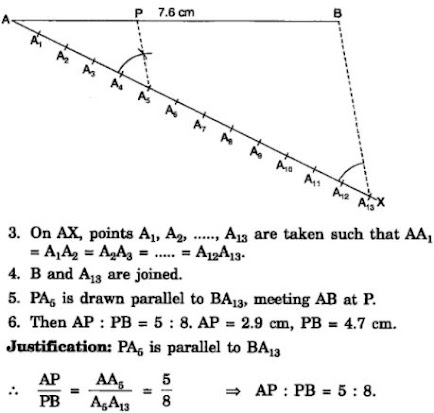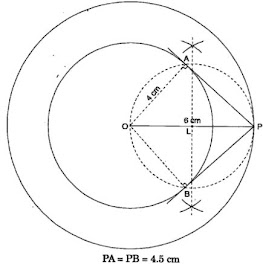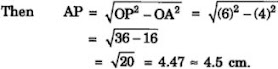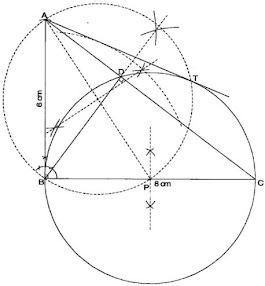# NCERT Solution for Class 10 Mathematics Chapter 11 Constructions

 Chapter Name NCERT Solution for Class 10 Maths Chapter 11 Constructions Topics Covered Short Revision for the ChapterNCERT Exercise Solution Related Study NCERT Solution for Class 10 MathsNCERT Revision Notes for Class 10 MathsImportant Questions for Class 10 MathsMCQ for Class 10 MathsNCERT Exemplar Questions For Class 10 Maths

## Short Revision for Constructions

1. The bisector  of an angle passes between the two arms of the angles.
2. Each point on the bisector of any angle is equidistant from the arms of the angle.
3. in the process of constructing the perpendicular bisector of a line segment, the radius of each arc must be greater than the half of the line segment.
4. Each point on the perpendicular bisector of a line segment is equidistant from the end points of the line segment.
5. To construct a triangle when the sum of the three sides and the two base angles are given, first we draw the line segment consisting the sum of the three sides and the two base angles.

### NCERT Exercise Solutions

#### Exercise 11.1

In each of the following, give the justification of the construction also:
1. Draw a line segment of length 7.6 cm and divide it in the ratio 5 : 8. Measure the two parts.

Solution

Steps of construction :
1. A line AB of length 7.6 cm is drawn.
2. Any angle BAX is drawn.2. Construct a triangle of sides 4 cm, 5 cm and 6 cm and then a triangle similar to it whose sides are 2/3 of the corresponding sides of the first triangle.

3. Construct a triangle with sides 5 cm, 6 cm and 7 cm and then another triangle whose sides are 7/5 of the corresponding sides of the first triangle.

4. Construct an isosceles triangle whose base is 8 cm and altitude 4 cm and then another triangle whose sides are 3/2 times the corresponding sides of the isosceles triangle

Solution
Steps of construction:

5. Draw a triangle ABC with side BC = 6 cm, AB = 5 cm and ∠ABC = 60°. Then construct a triangle whose sides are 3/4 of the corresponding sides of the triangle ABC.

6. Draw a triangle ABC with side BC = 7 cm, ∠ B = 45°, ∠ A = 105°. Then, construct a triangle whose sides are 4/3 times the corresponding sides of ∆ ABC.

7. Draw a right triangle in which the sides (other than hypotenuse) are of lengths 4 cm and 3 cm. Then construct another triangle whose sides are 5/3 times the corresponding sides of the given triangle.

Solution

Steps of construction :
(i) Construct the right triangle ABC such that ∠B = 90° , BC = 4 cm and BA = 3 cm.#### Exercise 11.2

In each of the following, give the justification of the construction also:

1. Draw a circle of radius 6 cm. From a point 10 cm away from its centre, construct the pair of tangents to the circle and measure their lengths.

Solution

Steps of construction :
1. A circle of radius 6cm is drawn.2. A point P is taken outside the circle such that OP = 10 cm.
3. Perpendicular bisector of OP is drawn, meeting OP at L.
4. With L as centre and OL as radius a circle is drawn, meeting the given circle at A and B.
5. PA and PB are joined.
6. PA and PB are the required tangents PA = 8 cm = PB.

2. Construct a tangent to a circle of radius 4 cm from a point on the concentric circle of radius 6 cm and measure its length. Also verify the measurement by actual calculation.

Solution

Steps of construction :
1. Two concentric circles of radii 4 cm and 6 cm are drawn.
2. P is a point on the circle with radius 6 cm.
3. Perpendicular bisector of OP is drawn, meeting OP at L.
4. With L as centre and OL as radius a circle is drawn, meeting the circle with radius 4 cm at A and B.5. PA and PB are joined.
6. PA and PB are the required tangents, such that PA = 4.5 cm = PB.
Calculation :
In △AOP, OA = 4 cm and OP = 6 cm3. Draw a circle of radius 3 cm. Take two points P and Q on one of its extended diameter each at a distance of 7 cm from its centre. Draw tangents to the circle from these two points P and Q

4. Draw a pair of tangents to a circle of radius 5 cm which are inclined to each other at an angle of 60°.

Solution

Steps of construction :
1. A circle with centre O of radius 5 cm is drawn.
2. Taking a radius OB as base and O as centre, an angle of measure 120° is constructed. So ∠AOB = 120° .
3. Tangents AP and BP are drawn to the circle at A and B, meeting each other at P.
4. Then PA and PB are the required tangents, such that ∠APB = 60° .5. Draw a line segment AB of length 8 cm. Taking A as centre, draw a circle of radius 4 cm and taking B as centre, draw another circle of radius 3 cm. Construct tangents to each circle from the centre of the other circle.

Solution

Steps of Construction :
1. A line segment AB = 8 cm is drawn.2. With A as centre a circle of radius 4 cm is drawn and with B as centre a circle of radius 3 cm is drawn.
3. With L as centre and AL as radius a circle is drawn meeting smaller circle at S' and T' and bigger circle at S and T.
4. AS', AT' and BS, BT are joined. Then AS' , AT' , BS, BT are the required tangents.

6. Let ABC be a right triangle in which AB = 6 cm, BC = 8 cm and ∠ B = 90°. BD is the perpendicular from B on AC. The circle through B, C, D is drawn. Construct the tangents from A to this circle.

Solution

Steps of construction :
1. A right - angled triangle ABC is drawn such that AB = 6 cm, BC = 8 cm, ∠ABC = 90° .
2. BD is drawn perpendicular to AC, meeting AC at D.3. ∠BDC = 90° , therefore, BC is hypotenuse. Hence, perpendicular bisector of BC is drawn to find mid - point P.
4. A circle through B,C, D is drawn, such that BC is diameter and P is its centre.
5. AP is joined.
6. A circle is drawn with AP as diameter meeting the circle at B and T.
7. AT is joined.
8. Then AB and AT are the required tangents.

7. Draw a circle with the help of a bangle. Take a point outside the circle. Construct the pair of tangents from this point to the circle.

Solution

Steps of construction :
(i) Draw the given circle using a bangle.
(ii) Take two non parallel chords PQ and RS of this circle.
(iii) Draw the perpendicular bisectors of PQ and RS such that they intersect at O. Therefore, O is the centre of the given circle.
(iv) Take a point P outside this circle.
(v) Join OP and bisect it. Let M be the mid point of OP.
(vi) Taking M as centre and OM as radius, draw a circle. Let it intersect the given circle at A and B.
(vii) Join PA and PB. Thus, PA and PB are the required two tangents.